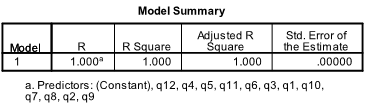# Jeromy Anglim's Blog: Psychology and Statistics

## Wednesday, May 13, 2009

### Verifying How Composite Scores Have Been Computed

This post sets out a procedure for checking how a composite was computed from items. This is particularly useful when working with self-report psychological scales and composite ability tests. Computing total scores for a series of subscales and total scores on personality tests and other self-report inventories can be fiddly business, and errors often arise.

The reason for wanting to check the method of computing the composite may be because:
• You want to verify that the composite was created correctly
• You are returning to the dataset after a long hiatus and have forgotten exactly how it was computed
• The composite was computed by someone else
For example, you might have a question with 20 or more items and:
• these items are used to calculate two or more subtotals each based on a subset of items
• the scores and subtotals may be based on a set of items after some items have been removed
• some items may have been reversed
• you may have made a decision about whether to use a total or a mean score.
GENERAL PROCEDURE
A useful way to check that the total scores have been computed correctly is to run a multiple regression with the total score as the dependent variable and the items as the predictor variables. If you have put the correct items in and total is correctly computed, r-square should be at or close to 1.00 and the regression coefficients should be 1.00 for included items (-1.00 for reversed items) and close to 0 for excluded items (often you will see values like 1.2E-5, which is basically the same as zero). If you have computed a mean for your composite instead of a total, then instead of the regression coefficients for included items being 1.00, they should be 1/k, where k is the number of items (e.g., for 5 items, each regression coefficient should be 1/5 = 0.20).
Let’s look at some examples.

Example 1.
Take a 12 item scale.In SPSS I computed a total score based on all items.
COMPUTE qtot=mean(q1 to q12)*12.

In SPSS Press: Analyze : Regression : Linear
Independents = all 12 items
Dependent = Total ScoreNote how the r-squared is 1.00 and the unstandardised regression coefficients are all 1.00.

This reflects the fact that:
TOTAL = 1*q1 + 1*q2 + … + 1*q12

Example 2.
Once again imagine a scale with 12 items. This time imagine that you have forgotten how the total was calculated. You are pretty sure that some items were reversed and some excluded, but which ones were they.

Analyze : Regression : Linear
Independents = all 12 items
Dependent = Total ScoreThe above table shows that the total is a function of the items (R-squared = 1.00).
Items 2 and 3 were reversed (see the -1.00 unstandardized coefficients)
Items 1, 9, 11, and 12 were excluded (see the unstandardised coefficients are all essentially zero).
Thus, if you need to check how a composite was coded, a multiple regression is a useful tool.
It is also good advice to store as syntax any commands that compute composite scores.

If you don’t get an r-squared of 1, there are many possible explanations: items have been left out of the composite; something was problematic with your method of computing the composite; etc.